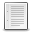File : Details

# partfrac()

Partial fraction decomposition of a rational in the C set
Details
Version
1.0
A more recent valid version with binaries for Scilab exists: 1.3.1
Author
Samuel Gougeon
Maintainer
Samuel Gougeon
Category
Supported Scilab Version
5.5
Creation Date
August 22, 2015Description
```            --> partfrac

Partial fraction decomposition of a rational in the C set

SYNTAX
------
partfrac    // Displays this help
i       = partfrac(f)
[i,r]    = partfrac(f)
[i,r,d]  = partfrac(f)
[i,r,d,t]= partfrac(f)

PARAMETERS
----------
f : Single rational = polynomial fraction with real or complex coefficients.
i : Integer part = non-fractional part of f (single polynomial).
r : Remainder of f: rational with degree(numerator) < degree(denominator)
d : Vector of rationals: rational Decomposition of r, in the complex set C,
such that sum(d)-r == 0. Denominators are all of degree 1.
t : 3-rows column of Texts. write(%io(2),t) displays i+d in a comprehensive
way, expliciting the multiplicity of poles in d. Coefficients format is
set with format().

DESCRIPTION
-----------
From a single polynomial fraction f = p/q with coprime polynomials p and q,
partfrac(f) extracts
* the non fractional part i of f: polynomial such that
0 <= degree(i) <= degree(p) - degree(q): i = p - modulo(p,q)
* the fractional part of f, or remainder r of p/q : r = modulo(p,q)/q
such that degree(numer(r))< degree(q) and  f = i + r
* the rational decomposition of r, aka partial fraction decomposition of f:
vector d of elementary rationals c/(x-pole)^m where c and poles are
decimal or complex numbers and m are the multiplicities of poles.

In addition, partfrac() may return as text the literal expression t of the
whole decomposition of f. This form shows the factorized forms of denominators
where poles that are multiple appear with their powers, while in d
denominators (x-pole)^(m>1) are developed. write(%io(2), t) may be used to
display it.

DEPENDENCY: If d or/and t is expected, polyroots() is required (>See also)

FEEDBACK
--------
Comments, scoring and bug reports are welcome on
http://fileexchange.scilab.org/toolboxes/451000#new_comment

--------
modulo    : remainder after polynomial division
polyroots : http://fileexchange.scilab.org/toolboxes/362000
pdiv_inc  : http://fileexchange.scilab.org/toolboxes/449000

EXAMPLE
-------
x = poly(0,"x");
f = (3-x+x^2-4*x^3+2*x^4) / ((x-1)*(x-2)^2)
[E, R, F, T] = partfrac(f);
E,R,F,T
write(%io(2),T)
clean(R-sum(F))

RESULTS
-------
--> x = poly(0,"x");

--> f = (3-x+x^2-4*x^3+2*x^4) / ((x-1)*(x-2)^2)
f  =
2    3    4
3 - x + x - 4x + 2x
-------------------
2   3
- 4 + 8x - 5x + x

--> [E, R, F, T] = partfrac(f);

--> E,R,F,T
E  =
6 + 2x

R  =
2
27 - 41x + 15x
--------------
2   3
- 4 + 8x - 5x + x

F  =
!     14            5             1     !
!  ---------  --------------  --------- !
!                          2            !
!  -2 +  1x    4 - 4x +  1x   -1 +  1x  !

T  =
!          14       5        1    !
!6 + 2x + ---- + -------- + ----  !
!         -2+x   (-2+x)^2   -1+x  !

--> write(%io(2),T)
14       5        1
6 + 2x + ---- + -------- + ----
-2+x   (-2+x)^2   -1+x

--> clean(R-sum(F))

0
-
1
```Files (2)Screenshot
```Screenshot of example for thumbnail
```Miscellaneous file
```File defining the function partfrac(), help contents and examples

* MAKE partfrac() AVAILABLE in EVERY SESSION: Click here to see HOW TO

* Get help: Enter "partfrac" without parameters
```News (0)Comments (0)